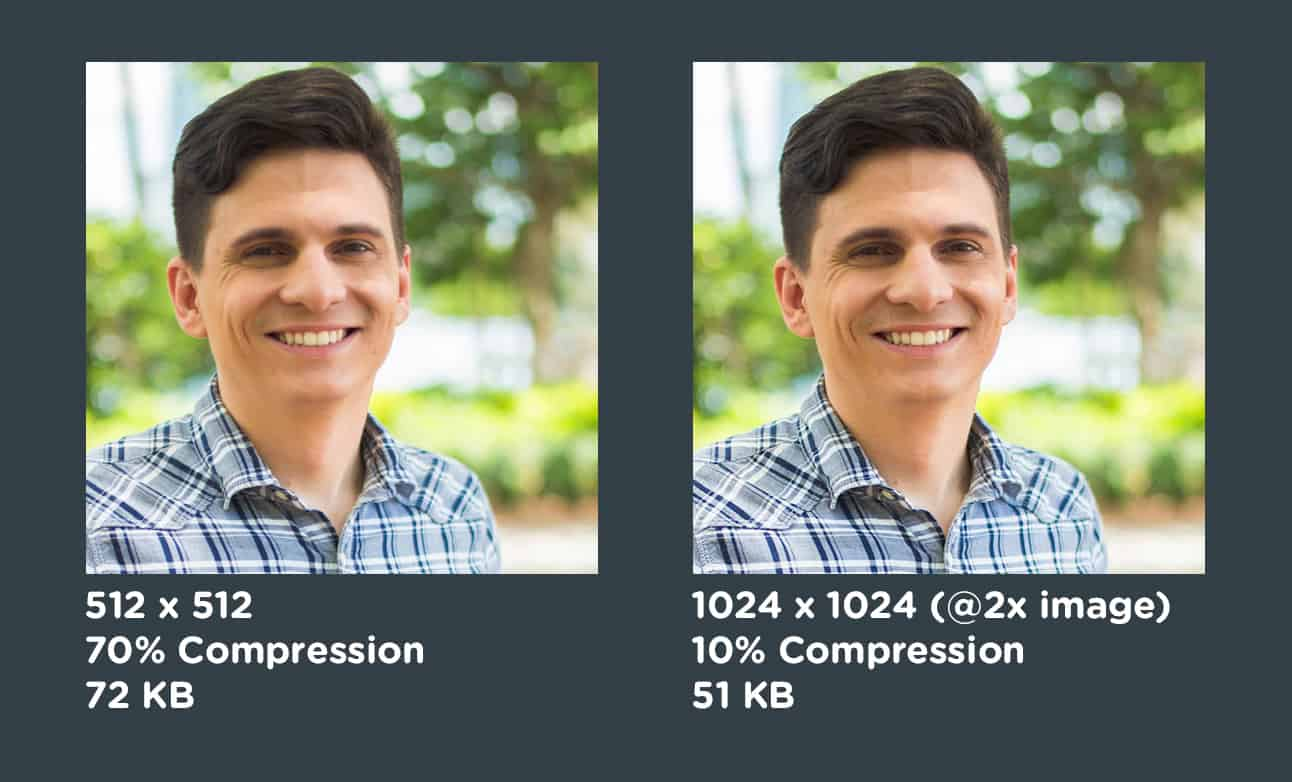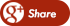﻿ how to do Image Compression in MATLABBack

## how to do Image Compression in MATLABImage Compression

Image  compression address the problem of reducing the amount of data required to  represent a digital image with no significant loss of information. Interest in image compression dates back more than 25 years. The field is now poised significant growth through the practical application of the theoretic work that began in 1940s , when C.E. Shannon and others first formulated the probabilistic view of information and its representation , transmission and compression.

Images  take a lot of storage space:

- - 1024 x 1024 x 32 x bits images requires 4 MB

- - suppose you have some video that is 640 x 480 x 24 bits x 30 frames per second , 1 minute of video would require 1.54 GB

Many  bytes take a long time to transfer slow connections – suppose we have 56,000  bps

- -  4MB will take almost 10 minutes

- - 1.54 GB will take almost 66 hours

Storage  problems, plus the desire to exchange images over the Internet, have lead to a  large interest in image compression algorithms.

The same information can be represented many ways.

Data  are the means by which information is conveyed. Various amounts of data can be used to convey the same amount of information. Example: Four different  representation of the same information ( number five) 1) a picture ( 1001,632 bits ); 2) a word  “five” spelled in English using the ASCII character set ( 32 bits); 3) a single ASCII digit ( 8bits); 4) binary integer ( 3bits)

Compression algorithms remove redundancy

If  more data are used than is strictly necessary, then we say that there is  redundancy in the dataset.

Data redundancy is not abstract concept but a mathematically quantifiable entity . If n1 and nc denote the number of information carrying units in two data sets that represent the same information, the relative data redundancy RD of the first data set ( n1 ) can be defined as

RD= 1 – 1/CR                                                                    (1)

Where CR is compression ration, defined as    CR = n1/nc                    (2)

Where n1 is the number of information carrying units used in the uncompressed dataset and nc is the number of units in the compressed dataset. The same units should be used for n1 and nc; bits or bytes are typically used.

When  nc<R® large  value and RD® 1. Larger  values of C indicate better compression

A general algorithm for data compression and image reconstruction

Input image ( f(x,y)                                                                                                 Reconstructed image f’(x,y)

An input image is fed into the encoder which creates a set of symbols from the input data. After   transmission over the channel, the encoded representation is fed to the decoder, where a reconstructed output image f’(x,y) is generated . In general , f’(x,y) may or may not an exact replica of f(x,y). If it is , the system is error free or information preserving, if not, some level of distortion is present in the reconstructed image .

Data compression algorithms can be divided into two  groups :

1 Lossless algorithms remove only redundancy present in the data . The reconstructed image is identical to the original , i.e., all af the information present in the input image has been preserved by compression .

2. Higher compression is possible using lossy algorithms which create redundancy (by discarding some information ) and then remove it .

Fidelity criteria:

When lossy compression techniques are employed, the decompressed image will not be identical to the original image. In such cases , we can define fidelity criteria that measure the difference between this two images. Two general classes of criteria are used : (1) objective fidelity criteria and (2) subjective fidelity criteria

A good example for (1) objective fidelity criteria is root-mean square ( RMS ) error between on input and output image For any value of x,and y , the error e(x,y) can  be defined as :

e(x,y) = f’(x,y) – f(x,y)Types of redundancy:

Three basic types of redundancy can be identified in a single image:

Coding redundancy

Interpixel redundancy

Psychovisual redundancy

Coding redundancy:

our quantized data is represented using codewords

The codewords are ordered in the same way as the intensities that they represent; thus the bit pattern 00000000, corresponding to the value 0, represents the darkest points in an image and the bit pattern 11111111, corresponding to the value 255, represents the brightest points.

if the size of  the codeword is larger than is necessary to represent all quantization levels,  then we have coding redundancy

An 8-bit coding scheme has the capacity to represent 256 distinct levels of intensity in an image . But if there are only 16 different grey levels in a image , the image exhibits coding redundancy because it could be represented using a 4-bit coding scheme. Coding redundancy can also arise due to the use of fixed-length codewords.

Grey level histogram of an image also can provide a great deal of insight into the construction of codes to reduce the amount of data used to represent it .

Let us assume, that a discrete random variable rk   in the interval (0,1) represents the grey levels of an image and that each rk occurs with probability Pr(rk). Probability can be estimated from the histogram of an image using

Pr(rk) = hk/n for k = 0,1……L-1                        (3)

Where L is the number of grey levels and hk is the frequency of occurrence of grey level (the number of times that the kth grey level appears in the image) and n is the total number of the pixels in the image. If the number of the bits used to represent each value of rk is l(rk), the average number of bits required to represent each pixelCoding redundancy – Example

Using eq. (2) the resulting compression ratio Cn is 3/2.7 or 1.11  Thus approximately 10 percent of the data resulting from the use of code 1 is redundant. The exact level of redundancy is

RD = 1 – 1/1.11 =0.099

Interpixel redundancy:

The intensity at a pixel may correlate strongly with the intensity value of its neighbors.

Because the value of any given pixel can be reasonably predicted from the value of its neighbors Much of the visual contribution of a single pixel to an image is redundant; it could have been guessed on the bases of its neighbors values.

We  can remove redundancy by representing changes in intensity rather than absolute  intensity values .For example , the differences between adjacent pixels can be used to represent an image . Transformation of this type are referred to as mappings. They are called reversible if the original image elements can be reconstructed from the transformed data set.

For example the sequence (50,50, ..50) becomes (50, 4).

Psychovisual redundancy:

Example First we have a image with 256 possible gray levels . We can apply uniform quantization to four bits or 16 possible levels The resulting compression ratio is 2:1. Note , that false contouring is present in the previously smooth regions of the original image.

The significant improvements possible with quantization that takes advantage of the peculiarities of the human visual system . The method used to produce this result is known as improved gray-scale ( IGS) quantization. It recognizes the eye’s inherent sensitivity to edges and breaks them up by adding to each pixel a pseudo-random number, which is generated from the order bits of neighboring pixels, before quantizing the result.